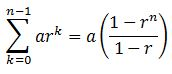# The Sum of the First n Terms of a Geometric Sequence

An error occurred trying to load this video.

Try refreshing the page, or contact customer support.

Coming up next: Understand the Formula for Infinite Geometric Series

### You're on a roll. Keep up the good work!

Replay
Your next lesson will play in 10 seconds
• 0:01 A Geometric Sequence
• 1:21 The Sum Formula
• 2:18 Using the Sum Formula
• 3:19 Another Example
• 4:11 Lesson Summary

Want to watch this again later?

Timeline
Autoplay
Autoplay
Speed

#### Recommended Lessons and Courses for You

Lesson Transcript
Instructor: Yuanxin (Amy) Yang Alcocer

Amy has a master's degree in secondary education and has taught math at a public charter high school.

Watch this video lesson to learn how you can find the total of a geometric sequence up to a certain point. Learn the formula that you can use to help you find your answer.

## A Geometric Sequence

In this video lesson, we are going to talk about geometric sequences and how to find the total of a geometric sequence up to a certain point. We call this the sum of the first n terms of a geometric sequence. Recall that a geometric sequence is a sequence where each term or number is the previous term or number multiplied by a certain constant. Each sequence has its own constant of multiplication, or common ratio.

An example of a geometric sequence happening in real life could be when you're growing potatoes. Suppose every potato you plant will give you 20 potatoes when it's done growing. And if you plant each of those 20 potatoes, you will get 20 new potatoes from each of those potatoes. So you end up with 20 * 20, or 400 potatoes. And if you plant these 400 potatoes, you will end up with 400 * 20 more potatoes.

For this potato example, our geometric sequence is looking like 1, 20, 400, and so on. Each number is the previous number multiplied by a certain constant, in our case 20. If you are the farmer, you might want to know how many potatoes you have grown over time. You want to add up all the potatoes you have grown over time. This is when you would use our handy formula for finding the sum of the first n terms of a geometric sequence.

## The Sum Formula

This formula begins with the sigma notation, which tells you that you will be adding up a series of numbers. Next comes the formula for finding each term of our geometric sequence. This part is simply a times r to the kth power. Our sigma notation tells us to add these terms, starting with k = 0 all the way up to n - 1.

All of this is equal to a times 1 minus r to the nth power over 1 minus r. This tells us that we can use the part after the equal sign to find our sum of our first n terms. We don't even have to add the individual terms. This is very helpful if we want to find the sum of a large number of terms.In this formula, a stands for our beginning term or number, r is our common ratio, and n is the number of terms we want to find the sum for.

To unlock this lesson you must be a Study.com Member.

### Register to view this lesson

Are you a student or a teacher?

### Unlock Your Education

#### See for yourself why 30 million people use Study.com

##### Become a Study.com member and start learning now.
Back
What teachers are saying about Study.com

### Earning College Credit

Did you know… We have over 160 college courses that prepare you to earn credit by exam that is accepted by over 1,500 colleges and universities. You can test out of the first two years of college and save thousands off your degree. Anyone can earn credit-by-exam regardless of age or education level.